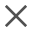Chat nowBasic Knowledge | 2019.07.19 | 1286
Thermal Resistance, Thermal Conduction, Thermal Conductance, Heat Transfer Resistance
Thermal Resistance is a heat property and a measurement of a temperature difference by which an object or material resists a heat flow.

Thermal Resistance is a heat property and a measurement of a temperature difference by which an object or material resists a heat flow.

The law of heat transfer can be compared to the “Ohm’s Law” that describes the law related to the electricity transfer in electrical science.

Ohm’s Law: I=U/R

where I is the electrical current flowing through the circuit; △V is the voltage drop between the two ends of the circuit and the electromotive force that drives the flow of electricity; R is the resistance of the resistor, representing the resistance in electricity transfer.

A similar formula in the process of plane heat transfer is: Φ=T/R where Φ is the amount of heat flow transferred; T is the temperature difference between the two ends and the force that drives the heat flow; R is the thermal resistance representing the resistance in heat transfer.

An object with better thermal conductivity generally has lower thermal resistance, measured in ℃/W or K/W.

Thermal Conduction is the reciprocal of thermal resistance, i.e. C=1/R，in W·m-2·K-1

Thermal Conductance, also known as heat transfer coefficient, refers to the quantity of heat that passes in unit time through an object of 1㎡ area and 1 m thickness when its opposite surfaces differ in temperature by 1K under steady state conditions. The legal measuring unit in China is W·m-1·K-1.

Heat Transfer Resistance is the inverse of heat transfer coefficient, measured in W-1·㎡·K. The greater the heat transfer resistance, the better the thermal insulation performance.